In this article we will compute weekly RSI (Relative strength Index) for stocks from daily stock data. Data from the API is in daily format. For longer swing trades or simply for long term investing entrypoints it is convenient to look on the bigger picture. Hence we would like our indicators to give us signals on weekly timeframe. Source of the data will be Yahoo! Finance API.

Main steps that will be performed:

• aggregate daily data to weekly time window
• compute RSI on the weekly data
• write simple RSI screening function to give buy/sell signals on weekly time frame

Import libraries

In :
from IPython.core.display import display, HTML
display(HTML("<style>.container { width:100% !important; }</style>"))
# ___library_import_statements___
import pandas as pd
# make pandas to print dataframes nicely
pd.set_option('expand_frame_repr', False)
import matplotlib.pyplot as plt
import datetime
import yfinance as yahoo_finance
%matplotlib inline


Choose a ticker and data date range

In :
# ___variables___
ticker = 'TSLA'                                               # Tesla stock

start_time = datetime.datetime(2017, 10, 1)
#end_time = datetime.datetime(2019, 1, 20)
end_time = datetime.datetime.now().date().isoformat()         # today


In :
# yahoo gives only daily historical data
def get_data(ticker, start_time, end_time):
connected = False
while not connected:
try:
df = web.get_data_yahoo(ticker, start=start_time, end=end_time)
connected = True
print('connected to yahoo')
except Exception as e:
print("type error: " + str(e))
time.sleep( 5 )
pass
return df

# run the function
df = get_data(ticker, start_time, end_time)

connected to yahoo

In :
# optional printing
# print(df.tail(7))


### Aggregate to weekly data¶

Resample daily data to weekly data and put it to new dataframe called r_df.

In :
# resample with dictionary
# each column has to be handled differently
agg_dict = {'Open': 'first',
'High': 'max',
'Low': 'min',
'Close': 'last',
'Volume': 'mean'}

r_df = df.resample('W').agg(agg_dict)


df is dataframe with daily data. r_df is resampled dataframe to weekly timeframe.

In :
# optional printing
# print('---------')
# print(r_df.tail(7))


### Visualize the data¶

Plot daily versus weekly price data.

In :
def plot_OHLC(data, ticker, window):

#to avoid matplotlib future warning
from pandas.plotting import register_matplotlib_converters
register_matplotlib_converters()

plt.figure(figsize=(15,5))
plt.title('{} price data to {} timeframe'.format(ticker, window))
plt.plot(data['Open'])
plt.plot(data['High'])
plt.plot(data['Low'])
plt.plot(data['Close'])
plt.legend()
plt.show()

In :
plot_OHLC(df, ticker, 'daily')
plot_OHLC(r_df, ticker, 'weekly')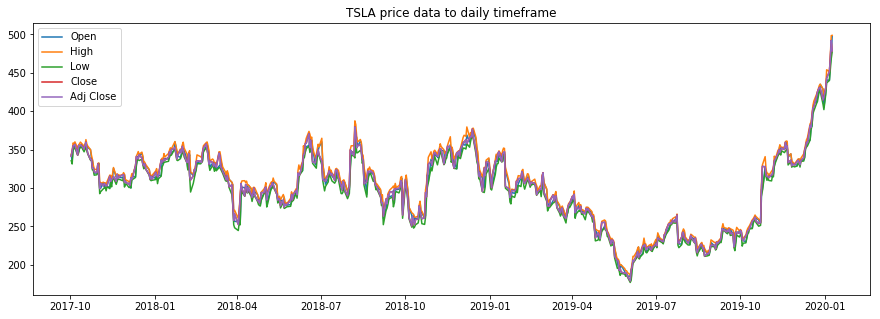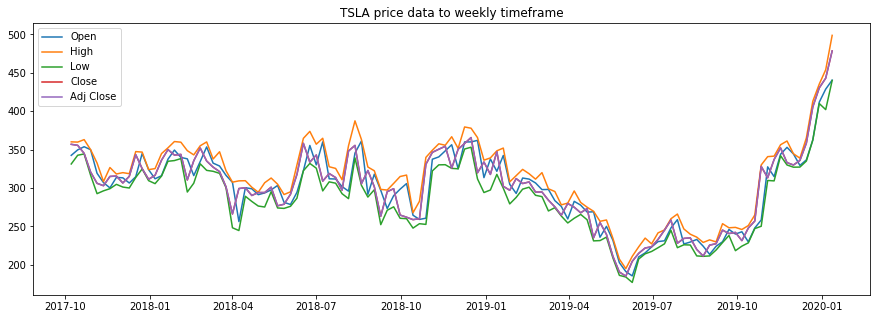Given a dataframe, compute RSI:

In :
def computeRSI(data, time_window):
diff = data.diff(1).dropna()        # diff in one field(one day)

#this preservers dimensions off diff values
up_chg = 0 * diff
down_chg = 0 * diff

# up change is equal to the positive difference, otherwise equal to zero
up_chg[diff > 0] = diff[ diff>0 ]

# down change is equal to negative deifference, otherwise equal to zero
down_chg[diff < 0] = diff[ diff < 0 ]

# check pandas documentation for ewm
# https://pandas.pydata.org/pandas-docs/stable/reference/api/pandas.DataFrame.ewm.html
# values are related to exponential decay
# we set com=time_window-1 so we get decay alpha=1/time_window

up_chg_avg   = up_chg.ewm(com=time_window-1 , min_periods=time_window).mean()
down_chg_avg = down_chg.ewm(com=time_window-1 , min_periods=time_window).mean()

rs = abs(up_chg_avg/down_chg_avg)
rsi = 100 - 100/(1+rs)
return rsi


Add newly computed RSI column to our daily and weekly dataframes.

In :
df['RSI'] = computeRSI(df['Adj Close'], 14)

In :
# optional printing
# print(df.tail())


Define RSI visualization function.

In :
def plot_price_and_RSI(dataframe, ticker, window):

# plot price
plt.figure(figsize=(15,5))
plt.title('{} price data (Adj Close) to {} timeframe'.format(ticker, window))
plt.show()

# plot corresponding RSI values and significant levels
plt.figure(figsize=(15,5))
plt.title('RSI chart')
plt.title('{} RSI chart to {} timeframe'.format(ticker, window))
plt.plot(dataframe['RSI'])

plt.axhline(0, linestyle='--', alpha=0.1)
plt.axhline(20, linestyle='--', alpha=0.5)
plt.axhline(30, linestyle='--')

plt.axhline(70, linestyle='--')
plt.axhline(80, linestyle='--', alpha=0.5)
plt.axhline(100, linestyle='--', alpha=0.1)
plt.show()


### Plot daily stock price and RSI¶

In :
plot_price_and_RSI(df, ticker, 'daily')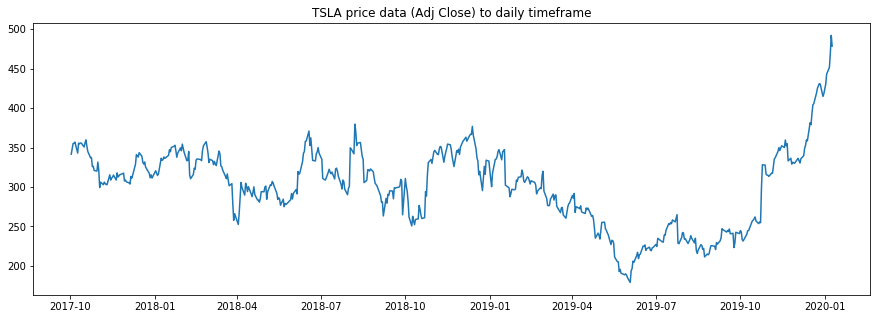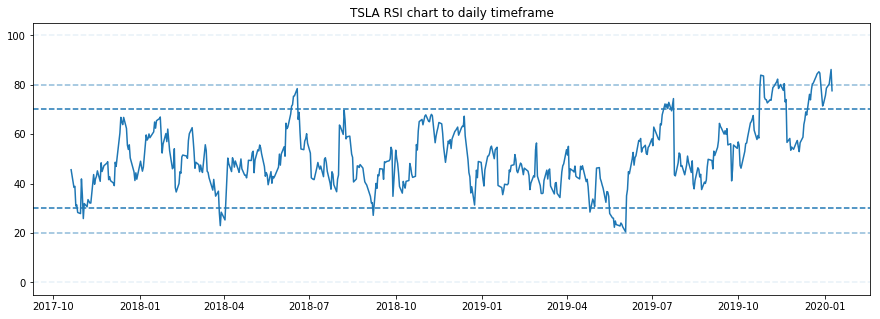### Plot weekly stock price and RSI¶

The weeklygraphs are the ones we are most interested in.

In :
plot_price_and_RSI(r_df, ticker, 'weekly')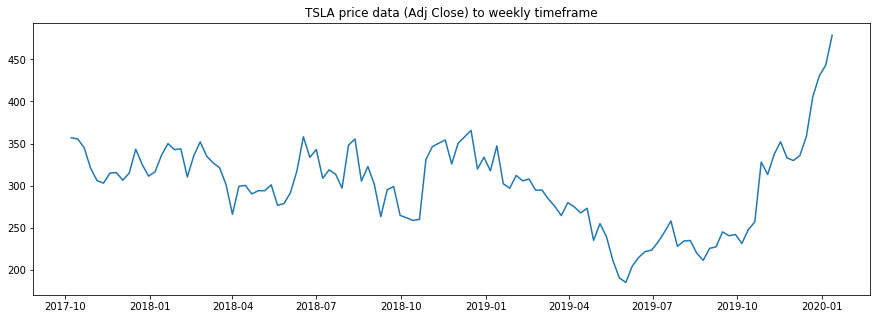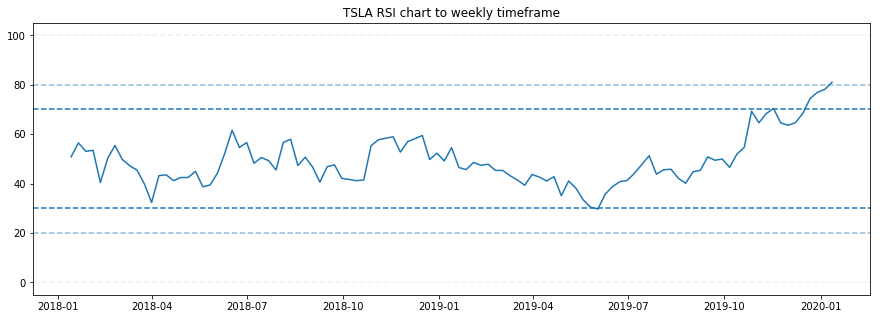#### Build simple stock buy/sell reccomendation system¶

Let's screen for some significant values of RSI that might give us actionable signals.

Now that we have weekly resampled RSI data in our dataframe, we can write super simple screener that would give us buy or sell signal based on the value of RSI.

• weekly RSI is > 70 ... sell signal
• weekly RSI is < 30 ... buy signal
In :
print('buy signal for below dates and prices based on RSI value')
print('--------------------------------------------------------')
print(r_df.loc[r_df['RSI'] < 30])

buy signal for below dates and prices based on RSI value
--------------------------------------------------------
Open   High         Low       Close   Adj Close    Volume        RSI
Date
2019-06-02  191.199997  195.0  184.100006  185.160004  185.160004  10153675  29.718747

In :
print('sell signal for below dates and prices based on RSI value')
print('---------------------------------------------------------')
print(r_df.loc[r_df['RSI'] > 70])

sell signal for below dates and prices based on RSI value
---------------------------------------------------------
Open        High         Low       Close   Adj Close    Volume        RSI
Date
2019-11-17  343.950012  356.329987  342.000000  352.170013  352.170013   7408020  70.414733
2019-12-22  362.549988  413.000000  362.500000  405.589996  405.589996  14730360  74.434420
2019-12-29  411.779999  435.309998  410.000000  430.380005  430.380005  10488475  76.934457
2020-01-05  428.790009  454.000000  402.079987  443.010010  443.010010  12545675  78.108997
2020-01-12  440.470001  498.799988  440.000000  478.529999  478.529999  18698713  81.034044


Rewrite screener into a function that will give us buy/sell signals:

In :
# r_df['RSI'].iloc[-1] means last/current RSI value
# the RSI value is for "today"

def scan_RSI(data, ticker):
print('Ticker:', ticker)

if r_df['RSI'].iloc[-1] > 70:
print('Current Weekly RSI:', r_df['RSI'].iloc[-1])
print('potential short/sell signal')
elif r_df['RSI'].iloc[-1] < 30:
print('Current Weekly RSI:', r_df['RSI'].iloc[-1])
else:
print('Current Weekly RSI:', r_df['RSI'].iloc[-1])
print('no current weekly RSI signals')



Call the function to to get buy/sell signal for current price.

In :
scan_RSI(r_df, ticker)

Ticker: TSLA
Current Weekly RSI: 81.03404442547773
potential short/sell signal


This function can be eventually part of stock sceening script.